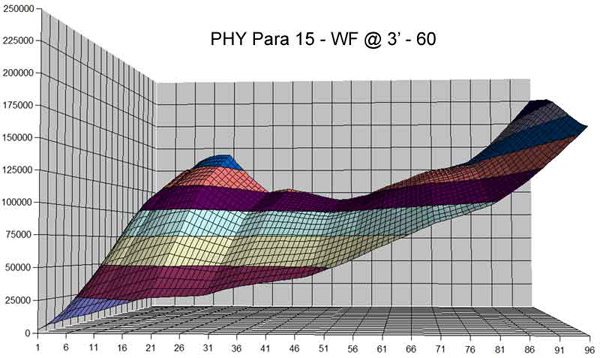WF vs DT Fly Line
 AFTMA Line Weights for first 30' of line not including the front level line.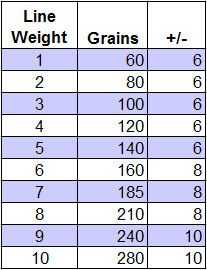The above is your basic AFTMA chart showing the standards for line weights. For example, the first 30' of a 5 weight fly line (not counting any level line before the beginning of the front taper) should weigh between 134 and 146 grains (8.7 to 9.5 grams).
 WF vs DT Fly Lines of equivalent tapers (Cortland 444 4wt)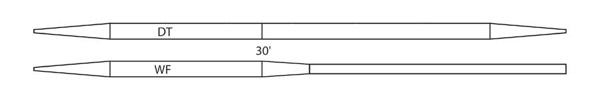The above illustration shows the basic taper anatomy of a 4wt Cortland 444 WF and DT fly line (detailed specs can be seen in a popup located below the next graphic). The important details to note are: The front tapers are identical. The front tapers begin and end at the same position on each fly line. The body on the DT line is the same diameter as the body on the WF line. In other words, the first 30' of both fly lines are absolutely identical in every way. Of course there are now many different tapers in fly lines.  You can have long front tapers, slow front tapers, half line weights, etc.  But, within the same fly line model and line weight, if you have a WF line and a DT line there is no difference at all when casting until you get more than 30' of fly line in the air.  And this does not include the level tip, leader or tippet. So for a typical ~40' cast there is effectively no difference between casting a WF or DT line (of equivalent weight and model).
 Calculated weights of a Cortland 444 fly lineAgain using the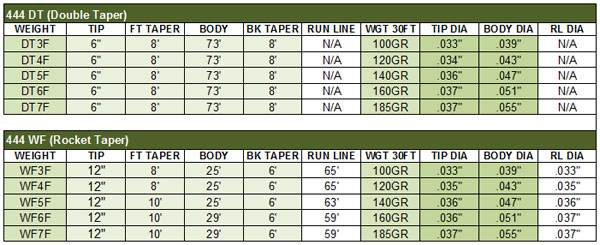specs from a Cortland 444 line, the above chart shows how much various lengths of line weigh in grains. As mentioned above, notice that up to 30' the WF and DT within each line class weighs exactly the same (There are slight variations due to where the tapers begin/end and small changes in the line's diameter).
 Graph showing calculated line weights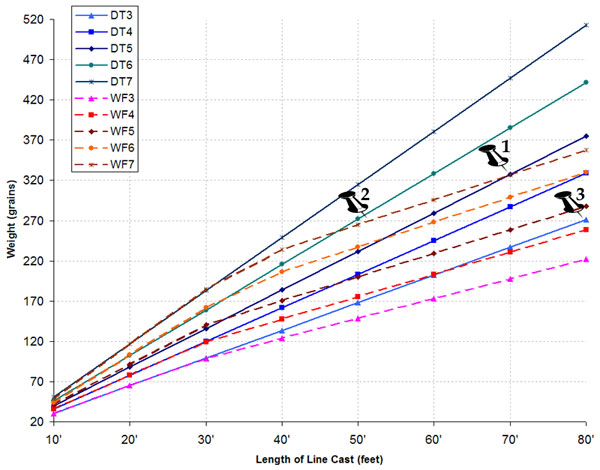Here is where things get interesting. The image above graphs the actual weight of each line type and line weight from the Calculated Weights Chart shown previously. DT lines are solid and WF lines are dashed. Notice at the 20-30' of the WF lines there is a distinct change in the angle of the graphed line. This designates the point where the rear taper on the WF lines occur. Now, if you look at the points that are pinned we see that: 70' of WF7 fly line weighs the same 70' of DT5 line. In other words, if you are making a 70' cast with a 5wt rod and a 5wt DT line, besides being impressive, the strain on your rod is the same as if you were casting the same distance but using a WF7 line. For a more common cast, roughly 47' of DT6 fly line weighs the same as 47' of WF7 fly line, so again, casting just under 50' of DT6 line exerts the same amount of strain on your fly rod as casting the same amount of WF7 line. For those monster casters, casting an entire DT4 fly line (80') is the same as casting an entire WF6 fly line (80'). The reason this is all so important in rod design, rod selection and line selection is one can use this to really dial-in how a rod performs at different casting distances. For example, one might want to use a WF fly line that is one line weight heavier than the rod designates because it loads the rod better in close, yet when you make longer casts, the rod won't overload as quickly as when using a DT line. Or, when making longer casts use a WF line to help keep from overloading the rod, or a DT if some extra line weight is needed to load the rod properly. This should help to dispel the myth that a DT line loads better in close than a WF line, or the tendency of some to rate a rod as, for example, a DT4/WF5. The only way this would make sense would be if one is rating the rod for casts longer than 30' of fly line (not including the line's level tip, the leader, or tippet).
 Stresses on a Para 15 at 3' to 60' (Z axis) of DT fly line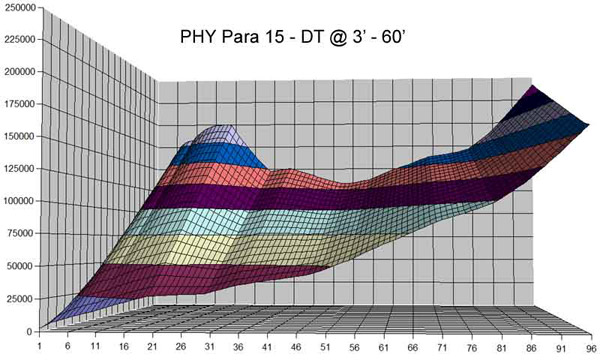The point of the above and below stress curve charts is to show the effect of the different line types. The Z-axis on the both charts equates to the amount of fly line in the air from 2' to 60', the Y-axis represents points along the rod with the tip on the left, and the Z axis is the stress on the rod during a cast.

On the lower graph, notice that approximately 2/3 of the way from the top - the point where the rear taper on a WF line starts - the rate of change of the rise of the stress decreases where the same point of the top graph continues to increase more-or-less consistently. By the time you get to the 60' point at the top of both charts, the bottom WF graph maxes out at ~140,000 and the top DT graph maxes out at ~200,000 translating to a roughly 30% increase in the stress on that part (~25" from the tip) of the rod when casting 60' of DT5 line vs 60' of WF5 line.
 Stresses on a Para 15 at 3' to 60' of WF fly line